Deep-dive

# Life in Differential Dataflow

Ruchir Khaitan

I’ve been working at Materialize for almost a year now, and I have really enjoyed learning about and using Differential Dataflow (hereafter just Differential) in my day-to-day work. In this post, I’ll introduce Differential and talk through implementing a few common programming problems like list intersection and everyone’s favorite, FizzBuzz, as dataflow programs. Finally, I’ll build a simple version of Conway’s Game of Life (hereafter just Life) in Differential. My main goal is to describe how to write algorithms in Differential and give some intuition for when that’s a good idea. This post requires some knowledge of Rust to read the examples but otherwise assumes no prior background. All the example code lives in a repository; you’ll just need to be able to build and run Rust programs to follow along!

Differential is a declarative, data-parallel, dataflow, incremental framework for performing computations over changing data. This is quite a jargon-y sentence so lets dress it down a bit.

• “Declarative” means that you don’t get to specify as much about how to implement an algorithm, vs what the algorithm does (and leave the how to the framework). In particular, you don’t get to make decisions like “should I use a hash table, binary tree or linked list here?” (a blessing and a curse). Instead, users are only allowed to think in terms of collections. Collections are generalized, multi-versioned multisets that store information in terms of `(data, time, multiplicity)` triples which means “at some discrete `time` we added `multiplicity` copies of `data` in our bag. `multiplicity` is allowed to be negative. Differential uses collections to represent changes over time, and thus positive multiplicities denote inserts and negative multiplicities denote deletes. If you’re still confused about collections there’s a more detailed explanation in the appendix.
• “Data-parallel” means the framework parallelizes computations by slicing and dicing data to different workers (when possible). Each worker gets a local copy of the data it needs and is then free to do its computation as if it were the only one. As the programmer you are free to write code and can be blissfully unaware of having multiple threads (most of the time).
• “Dataflow” means that you can’t think of your program as a single thread of control, where there are a sequence of instructions and some abstract-Turing-machine equivalent processor executes them one at a time1. Throw that model out. Instead, you have various functional operators, like `map`, `reduce` or `join` (among others). Each operator takes one or more collections as input, and spits out a new collection as output. All you can do to write programs is stitch these operators together into a dataflow graph by sending the outputs of some operators into the inputs of others. The actual code for this looks a lot like code you might write with iterators, except Differential lets you write loops where you connect some outputs back to earlier inputs. The similarity with iterators is deceptive, as all the Differential code we’ll write merely sets up the dataflows and their operators. A separate scheduler then invokes operators based on which ones have inputs that are ready to be used.
• “Incremental” means that as you send more data into your program, Differential will not recompute answers from scratch. Instead, Differential can re-use previously computed results to figure out new answers for changing inputs. In some cases, such as maintaining an average over a list of integers, this is already quite easy. In other cases, such as most graph algorithms, doing this by hand is quite hard. Differential dataflow makes this part automatic, and in my opinion, incremental computation is the most compelling reason to use Differential.

That’s a lot of words! Don’t worry if it doesn’t all make sense immediately it will (hopefully) get better as we dip our toes into some code.

## Intersecting Lists

Let’s start with a common interview problem: find the intersection (set of common elements) of two arrays of integers. This is probably one that most readers have seen before but still, let’s lay down a sketch of a fairly standard solution.

``````use std::collections::HashSet;

fn intersection(first: &[i32], second: &[i32]) -> Vec<i32> {
let mut output = Vec::new();

let first_set: HashSet<_> = first.iter().cloned().collect();
let second_set: HashSet<_> = second.iter().cloned().collect();

for element in first_set.iter() {
if second_set.contains(element) {
output.push(*element);
}
}

output
}
``````

There’s some minor Rust specific things here but by and large this should feel familiar to everyone who has written code in an imperative language. We take two arrays of integers as input, transform them both into sets, and then go through the integers in one set to see if they’re also in the second. If so, we add them to our output. Pretty simple. Let’s take a minute to appreciate two things: first, maintaining correct results over time while `first` and `second` changed arbitrarily would be hard, and second, we can’t reuse any of these pieces in Differential. We can’t convert arrays into hashsets because we only get collections, and we don’t have access to for loops because we don’t have access to control flow primitives.

Instead, we need to figure out a way to use dataflow operators. After digging around the docs for a bit, we can see that the semijoin operator looks very promising. `semijoin` takes two collections, one of type `(Key, Value)` and one of type `(Key)` and produces a collection of type `(Key, Value)` that contains all `(k, v)` pairs for keys with nonzero multiplicities in both collections. That’s not exactly the intersection we want but it’s awfully close. Unfortunately, we aren’t fully out of the woods yet. It’s clear that we can use `i32` as the key in both collections, but we don’t have any values. Thankfully, this is not a problem because we can use the unit type to simulate a value. We can use a `map` operator to turn a collection of type `T` into `(T, ())`. Similarly, we can use another `map` to convert back from type `(T, ())` to `T`. At this point, the output will be almost exactly what want, except that multiplicities in the output might be greater than one but we specifically want the intersection set. We can give the collection set semantics with the `distinct` operator. This implementation corresponds to the following dataflow graph.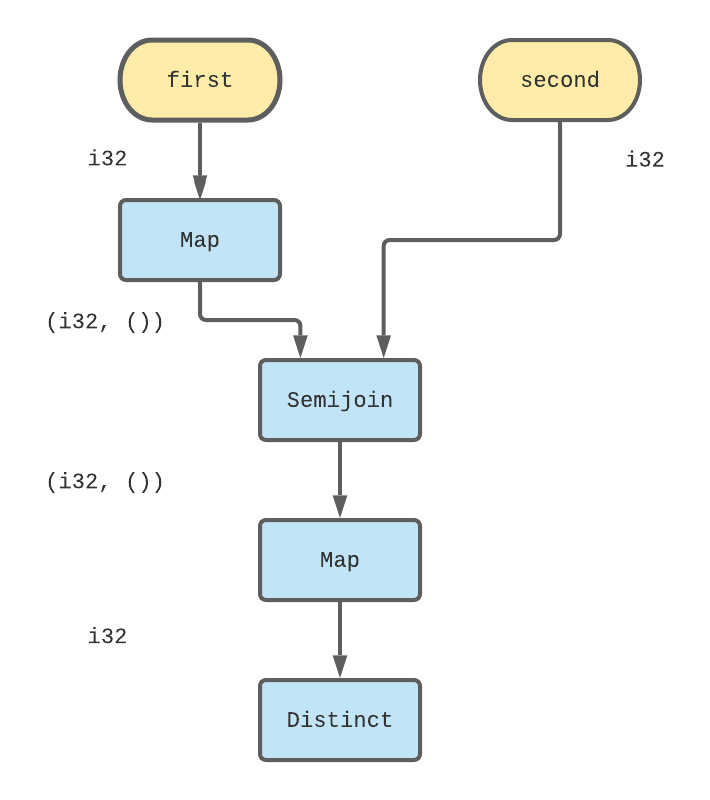Here, edges represent collections, and blue rectangles represent dataflow operators. I’ve labelled `first` and `second` with yellow ovals to indicate that they are input collections that get data from external inputs, rather than from another operator’s output. I’ve also annotated each collection with its type to make the intent of the `map`s more clear. The Differential version of this graph looks like the following code:

``````// Assume `first` and `second` are two input collections defined elswhere.
let output = first
.map(|x| (x, ()))
.semijoin(&second)
.map(|(x, _)| x)
.distinct();
``````

Before we go any further, I want to call out three things. First, this is one of many possible Differential solutions. For example, we could have used `distinct` on each of the inputs before we called `semijoin`. Alternatively, we could have used the `join` operator instead of the `semijoin` operator. All of these choices have various trade-offs that are unfortunately out of scope for this post but I want to highlight that it’s not as if Differential is so strongly “declarative” that there’s only one canonical way to express a program. Second, I want to be really explicit about the relationship between the visual dataflow graph and the actual code. Every operator (blue rectangle) corresponds to one of the operator function calls in the code. Every incoming edge corresponds to one of the arguments to those functions, and every outgoing edge corresponds to one of the outputs of those functions. Finally, and perhaps most importantly, we still don’t have the ability to send inputs to our dataflow operators and use all of this logic. We’ll need a bit more Differential and Timely (the underlying framework for distributed computation that Differential is built on) to get things going and the final result looks like:

``````timely::execute_directly(move |worker| {
let (mut first, mut second) = worker.dataflow(|scope| {
let (first_handle, first) = scope.new_collection();
let (second_handle, second) = scope.new_collection();
let output = first
.map(|x| (x, ()))
.semijoin(&second)
.map(|(x, _)| x)
.distinct();
output
.inspect(|(x, time, m)| println!("x: {} time: {} multiplicity: {}", x, time, m));
(first_handle, second_handle)
});

// Send some sample data to our dataflow
for i in 0..10 {

for x in i..(i + 10) {
first.insert(x);
second.insert(x + 5);
}
}
})
``````

We need to set up some Timely and Differential boilerplate here to get our computation going. We tell Timely to create a new dataflow graph with the `dataflow` method and can define our input collections with the `new_collection` method. `new_collection` gives us a “handle” which is basically like a pipe that we can use from elsewhere to send data into this collection, and a reference to the collection, that we can use within the closure to implement the actual graph (it’s the same logic as before). The only other novel bit in the closure is the `inspect` call which lets us print the contents of our collection to `stdout`.

The rest of the code outside of the closure deals with setting up an example. It’s sending integers to `first` and `second` at various logical times (based on when we `advance_to`) so that we can test out the logic. It’s not immediately obvious from the nested loop but `first` gets everything in `[0, 19)` (some of them repeated) and `second` gets everything in `[5, 24)` (again with repetitions). Therefore, the intersection set should be `[5, 19)` and indeed when I run this (you can see it too if you clone the repository) I see:

``````altaria-2:life-differential \$ cargo run --example intersection
Compiling life-differential v0.1.0 (/Users/Test/github/life-differential)
Finished dev [unoptimized + debuginfo] target(s) in 3.62s
Running `target/debug/examples/intersection`
x: 5 time: 0 multiplicity: 1
x: 6 time: 0 multiplicity: 1
x: 7 time: 0 multiplicity: 1
x: 8 time: 0 multiplicity: 1
x: 9 time: 0 multiplicity: 1
x: 10 time: 1 multiplicity: 1
x: 11 time: 2 multiplicity: 1
x: 12 time: 3 multiplicity: 1
x: 13 time: 4 multiplicity: 1
x: 14 time: 5 multiplicity: 1
x: 15 time: 6 multiplicity: 1
x: 16 time: 7 multiplicity: 1
x: 17 time: 8 multiplicity: 1
x: 18 time: 9 multiplicity: 1
``````

## FizzBuzz

I need to introduce one more operator before we can start working on Life, and like above, I’ll motivate it with a simple question: compute FizzBuzz for the numbers 1 – 100. An example solution is pretty simple.

``````for x in 1..=100 {
let str = if x % 3 == 0 && x % 5 == 0 {
"FizzBuzz"
} else if x % 5 == 0 {
"Buzz"
} else if x % 3 == 0 {
"Fizz"
} else {
""
};
println!("{} {}", x, str);
}
``````

This for-loop has a clear iterator `1..=100` that controls how many times the body of the loop executes. You could also choose to write it with a while loop like this:

``````let mut x = 1;
while x <= 100 {
let str = ... // Same if statement as above
println!("{} {}", x, str);
x = x + 1;
}
``````

Here, instead of directly specifying the number of iterations, I’ve specified a predicate that indicates when we should stop executing the loop. It’s a slightly different way to express the same idea. Differential has an operator for iteration called `iterate`2 but it doesn’t let you specify the iteration count, or a predicate to stop iterating. Instead, `iterate` repeatedly applies your logic (expressed as a dataflow fragment) to a collection until the output stops changing, aka reaches a fixed point. The process for writing dataflows like this feels less like writing a `for` loop, and more like writing an inductive proof. In that spirit I like to think of a partial result, and see what dataflow fragment would let us generate the next iterative result. More concretely, lets assume that we are storing our FizzBuzz data in a collection of type `(i32, String)` for simplicity and lets say that after four iterations, we have the following data:

``````(1, ""),
(2, ""),
(3, "Fizz"),
(4, ""),
``````

We’d like now to take that collection as input and produce everything from above + `(5, "Buzz")` as output. Paradoxically, trying to be clever here and trying to find the maximum integer generated so far or something like that isn’t going to be very helpful. Instead, we’ll try the simpler strategy of having every single element to produce its “successor” (e.g. we’ll transform `(2, "")` into `(3, "Fizz")`) and then combine the set of successors with the existing set of inputs. As long as we are careful to only retain one copy of everything, the resulting output should be what we want. The dataflow graph for this single iteration logic looks like: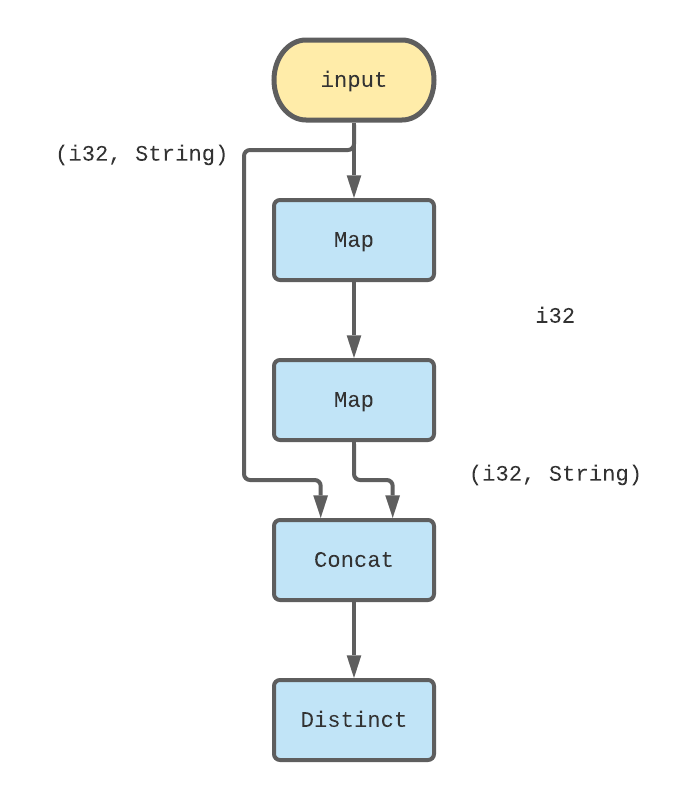The corresponding code for that logic looks like the following.

``````let successors = input.map(|(x, _)| x + 1).map(|x| {
let str = if x % 3 == 0 && x % 5 == 0 {
"FizzBuzz"
} else if x % 5 == 0 {
"Buzz"
} else if x % 3 == 0 {
"Fizz"
} else {
""
};

(x, str.to_string())
});
let output = input.concat(&successors).distinct();
``````

The second `map` ends up being exactly the same for-loop as in the imperative version! From here, we just need to encode logic that will make the collection stop at 100. Remember, we can’t control how many times the dataflow computation will execute but we can control what we emit as output. In this case, we can use a `filter` operator to restrict our FizzBuzz output to `[1, 100]` . We can now take our logic for handling a single iteration of FizzBuzz and use it as the logic for `iterate`. This is our final dataflow graph for FizzBuzz: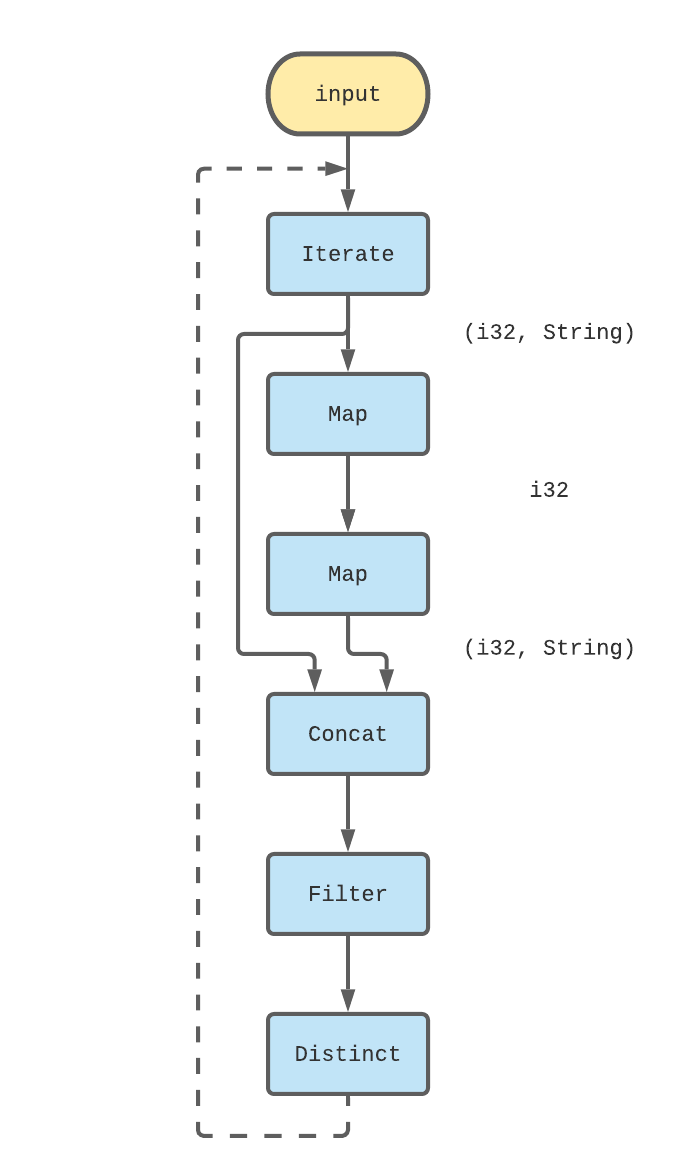The final Differential code for FizzBuzz should look familiar at this point.

``````timely::execute_directly(move |worker| {
worker.dataflow::<u32, _, _>(|scope| {
// Seed the iteration with (1, "")
let initial = scope
.new_collection_from(vec![(1, "".to_string())].into_iter())
.1;

let result = initial.iterate(|input| {
let successors = input.map(|(x, _)| x + 1).map(|x| {
let str = if x % 3 == 0 && x % 5 == 0 {
"FizzBuzz"
} else if x % 5 == 0 {
"Buzz"
} else if x % 3 == 0 {
"Fizz"
} else {
""
};

(x, str.to_string())
});
let output = input.concat(&successors).distinct();
output.filter(|(x, _)| *x <= 100)
});
result
.inspect(|(x, time, m)| println!("x: {:?} time: {:?} multiplicity: {}", x, time, m));
});
})
``````

You can run it by typing the following:

``````altaria-2:life-differential Test\$ cargo run --example fizzbuzz
Compiling life-differential v0.1.0 (/Users/Test/github/life-differential)
Finished dev [unoptimized + debuginfo] target(s) in 4.40s
Running `target/debug/examples/fizzbuzz`
x: (1, "") time: 0 multiplicity: 1
x: (2, "") time: 0 multiplicity: 1
x: (3, "Fizz") time: 0 multiplicity: 1
x: (4, "") time: 0 multiplicity: 1
x: (5, "Buzz") time: 0 multiplicity: 1
...
``````

There’s a few wrinkles in this code as well that are worth mentioning. First, note that its absolutely mandatory to seed this computation with a valid value. If we don’t do that, the iteration will stop because it will quickly reach a fixed point with the empty collection. Debugging cases like that is tricky and I still forget to properly do this from time to time. Also, having a `distinct` or `consolidate` or something else that forces exactly one instance of each `datum` at a particular `time` is also mandatory. Otherwise, we may end up with collections that alternate between states that are logically equivalent (in that the sum of all data’s multiplicities at each time are identical) but not physically equivalent (we haven’t actually done this addition), and then an otherwise convergent computation may not converge. Finally, I want to address the largest concern I had when first writing this code. This implementation, at first glance, seems like a quadratic time implementation of FizzBuzz (which probably wouldn’t pass most interviews). It certainly seems as if, at each iteration `i`, we do the work to generate all `i` FizzBuzz numbers again. Fortunately, this is not what happens because all of the operators are properly incremental and thus at each iteration `i` we only perform work proportional to the changes in the input between iterations `i - 1` and at `i`. Thus, in every iteration, we only generate one new element, and the overall work involved is strictly linear. Exactly how this works is a bit out of scope but you can get some of the details from the original paper.

Some people may not trust the explanation above. I certainly wasn’t sure of it myself initially. So let’s test this out. We can compile FizzBuzz in release mode, pipe the output away to `/dev/null` to make sure we’re not bottlenecked on printing to the terminal, run it on different input sizes with a command line argument and measure the results with `time`. The invocation looks something like:

``````altaria-2:life-differential \$ time cargo run --release --example faster-fizzbuzz -- 1000000 > /dev/null
``````

I ran each test a few times and picked a number that looked close to median. Not my most scientific benchmark 🙂

Input size Elapsed time (seconds)
1,000 0.11
10,000 0.48
100,000 4.31
1,000,000 44.05

However, the results are pretty clear. This program scales linearly with the input and it’s a pretty slow implementation. We don’t even technically need `iterate` to compute FizzBuzz, but in general, this approach is slow because we do so little new work per iteration. We can easily make this ~50x faster by changing the code to take a logarithmic number of iterations, where each iteration generates twice as many new values as the one before and being clever to avoid duplicates without using stateful operators like `distinct`. The code for all of those optimizations is here. Anyway, with that we’re ready to move on from FizzBuzz and work on implementing Life.

## Life

Let’s briefly touch on the rules. We have a (infinite) grid of square cells. All cells have 8 adjacent neighbors and all the cells are either “dead” or “live”, and the game evolves in discrete rounds as follows:

1. Any live cell with two or three live neighbors stays live in the next round. (moral of the story: you need to have friends but not too many)
2. Any dead cell with three live neighbors becomes live in the next round (this is how babies are made)
3. All other live cells die in the next round. All other dead cells stay dead in the next round. (some cells die of natural causes, some get killed by their neighbors; no zombies)

Let’s start by imagining how we might do this in an imperative language. We could, for example, use an array to store the cells, and use a doubly nested for-loop to iterate through cells and evolve their state over rounds. I won’t bother writing down a full Rust implementation because clearly, this won’t give us a lot of insight into how to express it in terms of a dataflow graph.

Instead, let’s make some some concrete decisions about how we want to represent this problem as collections and see if we can sketch out what we would need to do to make things work. I propose that we represent cells using pairs of integers `(x, y)` indicating their coordinates in the grid and furthermore, that we keep a collection of cells that are “live” at a round, and use `iterate` to evolve that collection over rounds. Unlike FizzBuzz, Life isn’t guaranteed to terminate. For now, let’s ignore that and revisit it later.

We’re in a position pretty similar to the one we were in earlier. We want to write a dataflow graph that can take in a set of pairs as input, and produce a new set of pairs as output. Unlike FizzBuzz, there isn’t a natural mapping from each input element to an output element. Instead, outputs depend on the numbers of live neighbors each cell has. In an imperative setting, we might write code that asks “how many of my neighbors were live?” for each cell in the grid. In Differential, we’ll have an easier time if we let each live cell announce “these are my neighbors!” and then count how many live cells each announced neighbor was adjacent to.

It’s hard to describe the idea fully in prose so let’s go through a visual example. Imagine that this is the state of our grid at some round, and the cells shaded in blue are live, and the rest are dead.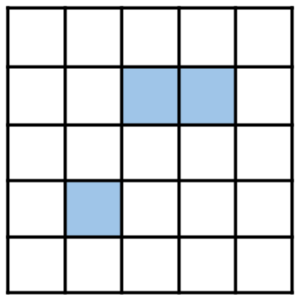We can visualize the potentially live cells in the next round as follows. Only filled in cells below had at least one live neighbor this round, and so they are the only ones who might be live in the next round. The cells shaded in light pink had exactly one live neighbor, the cells shaded in pink had two live neighbors, and the one cell in the very center that’s shaded in dark magenta has three live neighbors. That’s the only cell that will be live in the next round. The cells with blue borders represent the cells that were themselves live in the preceding round but unfortunately none of them had enough live neighbors to make it through to the next. We want to write a dataflow fragment that starts with the previously live cells (the blue cells from above), and gives potentially live cells (shaded cells below), before filtering down to only live ones in the next round.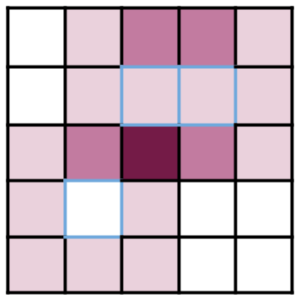First, we have to get each live cell to propose all of its neighbors. Fortunately, we can do that with a little bit of arithmetic.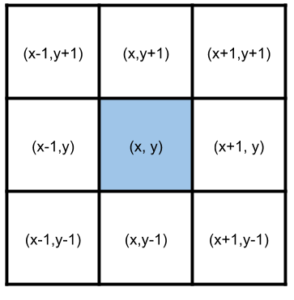We can use the `flat_map` operator to do this arithmetic and generate 8 neighbors from each live cell, and then we can `count` the number of times each neighbor was emitted (by a formerly live cell) to end up with a collection of potentially eligible cells and the number of live neighbors each of them had. I’ve added some English annotations on the right to go along with the type signatures of each collection on the left.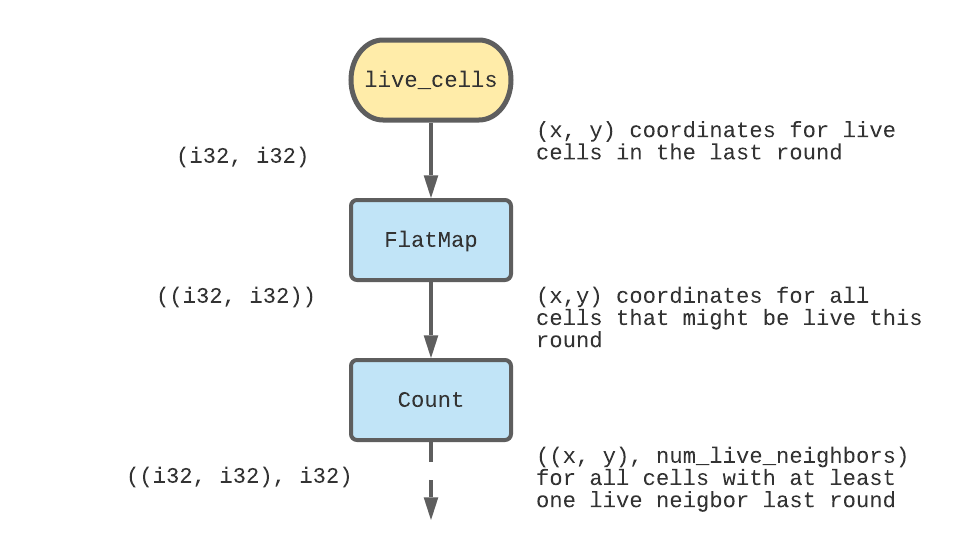The code for this part is:

``````let maybe_live_cells = live_cells.flat_map(|(x, y)| {
[
(-1, -1),
(-1, 0),
(-1, 1),
(0, -1),
(0, 1),
(1, -1),
(1, 0),
(1, 1),
]
.iter()
// This map is a function over an iterator, not a dataflow operator.
.map(move |(dx, dy)| ((x + dx, y + dy))
})
.count();
``````

Next, we need to figure out a way now to apply the evolution rules 1 – 3 above to this collection of `maybe_live_cells`. As written, those rules require us to know “was this cell live in the previous round” which we don’t currently have access to. But if we transpose rules 1 and 2 a little bit, we can rewrite them as:

• all cells that have 3 live neighbors are live in the next round.
• all cells that have 2 live neighbors and are currently live stay live in the next round.

This now lets us take action based on the data we have. We can filter out the set of cells with 3 live neighbors; all of these cells will be live in the next round. We can also filter out the set of cells with 2 live neighbors, and now `semijoin` them against the `live_cells` from before to figure out which of these were previously live. Finally, we can `concat` the two result collections together, and that’s the set of live cells in the next round! As with FizzBuzz, this logic describes a single iteration of Life, and we can place the whole thing inside of an `iterate` loop and that’s pretty much the full implementation.

Let’s take a step back and look at the dataflow graph I’ve only verbally described so far.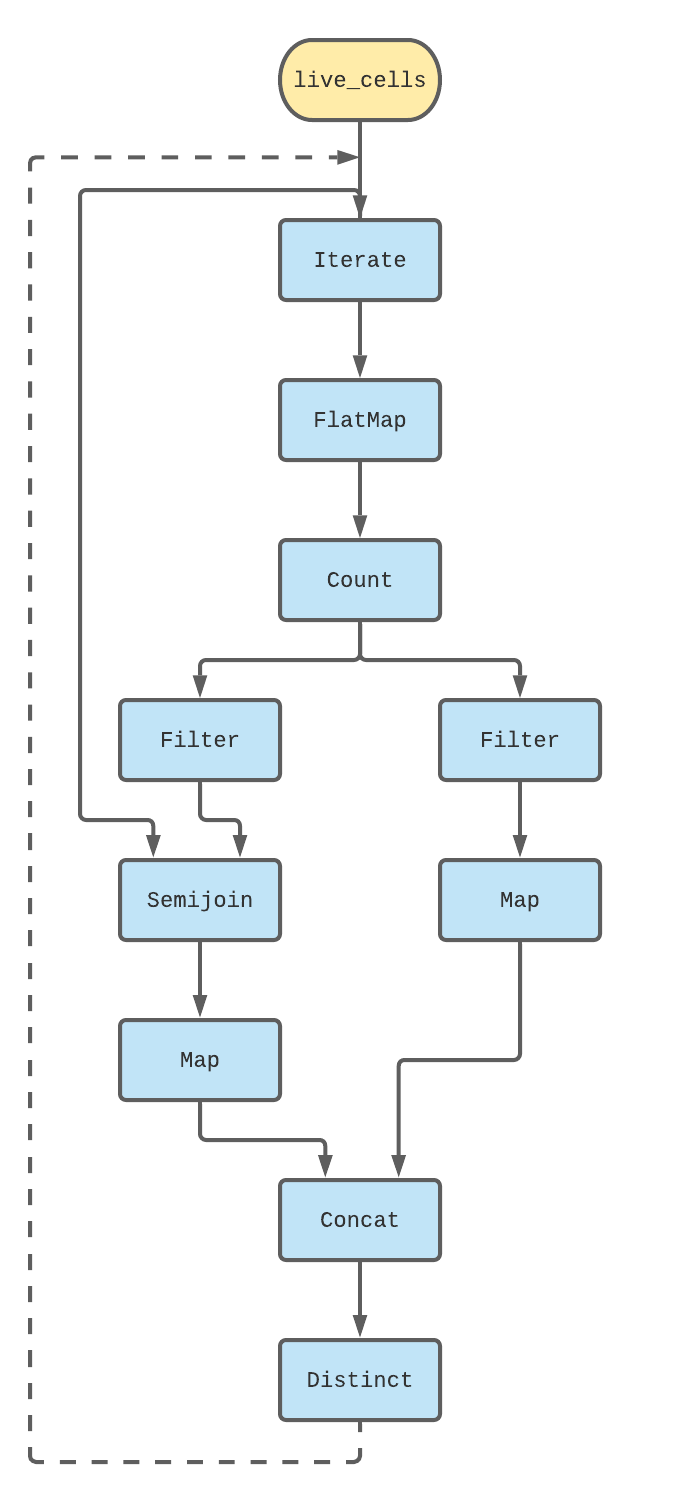The code for this snippet, which does the logic for Life, follows pretty closely:

``````live_cells.iterate(|live| {
let maybe_live_cells = live
.flat_map(|(x, y)| {
[
(-1, -1),
(-1, 0),
(-1, 1),
(0, -1),
(0, 1),
(1, -1),
(1, 0),
(1, 1),
]
.iter()
.map(move |(dx, dy)| ((x + dx, y + dy)))
})
.count();

let live_with_three_neighbors = maybe_live_cells
.filter(|(_, count)| *count == 3)
.map(|(cell, _)| cell);
let live_with_two_neighbors = maybe_live_cells
.filter(|(_, count)| *count == 2)
.semijoin(&live)
.map(|(cell, _)| cell);

let live_next_round = live_with_two_neighbors
.concat(&live_with_three_neighbors)
.distinct();

live_next_round
})
``````

And thats pretty much it! We can run this (the rest of the code in `src/main.rs` seeds the computation with a list of starting live cells so that Life converges in a few rounds).

``````altaria-2:life-differential \$ cargo run
Finished dev [unoptimized + debuginfo] target(s) in 0.16s
Running `target/debug/life-differential`
x: 1, y: 3, time: 0 diff: 1
x: 2, y: 2, time: 0 diff: 1
x: 2, y: 3, time: 0 diff: 1
x: 3, y: 2, time: 0 diff: 1
x: 1, y: 2, time: 0 diff: 1
x: 3, y: 3, time: 0 diff: 1
x: 2, y: 1, time: 0 diff: 1
x: 2, y: 2, time: 0 diff: -1
x: 2, y: 3, time: 0 diff: -1
x: 2, y: 4, time: 0 diff: 1
``````

It’s not the most thrilling graphics :). You can edit the list of starting cells to see more complex and infinitely evolving grids with fun things like gliders or other more exotic automata. While this is technically a working Game of Life implementation, it’s not very useful at the moment because we can’t ask it to evolve say, the next 20 iterations. It’s all or nothing and it won’t stop until the game state converges to a fixed point which might never happen. We’ll fix all of these things, and compare the Differential version’s performance against a more standard implementation in the next post. Also, we’ll show off some pretty mind-bending time travel-esque things Differential lets you do with partially ordered times that would be pretty hard to do by hand.

If you thought this post was cool and want to learn more about Differential, you should check out the mdbook. If you want to see an example of Differential being used in the real world you should check out the Materialize source code!

Thanks Andi, Eli, Frank, Justin, Matt and Paul for reading earlier versions of this post and providing valuable feedback.

## Appendix: Collections

Collections are time varying, generalized multisets3. A multiset is basically a relaxation of a set, where you can have multiple copies of each element. A grocery bag with 3 bananas, an apple, and two carrots is a multiset containing the set of foods `banana, apple, carrot`. In this grocery bag, the `multiplicity` of banana is `3`. In collections, multiplicities can be negative as well. In collections we can think of negative multiplicities as deletions, and positive multiplicities as inserts at various times.

Imagine we had a collection of fruits and vegetables. We’ll represent the updates going into the collection as 3-tuples of `(data, time, multiplicity)` where in this case `data` is a fruit or vegetable, `time` is a positive integer indicating logically when this data should be applied, and `multiplicity` is a positive or negative integer indicating how the multiplicity changed with this update. Note that `time`s can be partially ordered, but in this example they will be totally ordered.

For example, lets say we got the following three updates:

``````(potato, 0, 1)
(apple, 0, 1)
(potato, 0, 3)
``````

And if this were all of the updates at time 0, the final state of this collection as of time 0 would be:

``````(apple, 0, 1)
(potato, 0, 4)
``````

If at times 1 and 2 we get some more updates like

``````(kiwi, 1, 6)
(apple, 1, 1)
(banana, 1, -2)
(apple, 2, -2)
``````

Then the final state of the collection as of time 2 would be:

``````(banana, 2, -2)
(potato, 2, 4)
(kiwi, 2, 6)
``````

Despite my best efforts we still have `-2` bananas. This might seem weird but its actually just fine in Differential land. The nice thing about this representation and the flexibility with negative multiplicities is that its really easy to consolidate different updates to the same data over time.

1. Another way to think about it is that normal code lets us make decisions about which blocks of code execute before or after others. We don’t get to make decisions like that with dataflow code.
2. In fact you can compute FizzBuzz with just `flat_map` because there isn’t actually a dependency between one value and the next. But it’s a good and simple example :)
3. This isn’t exactly true anymore but is a useful mental model nonetheless. See this post for a more complex use case of collections that aren’t really multisets, but instead maps from `(data, time)` to instances of monoids.